• 应用统计主元分析例程，本例子提供例程，只需要将代码复制到Matlib中就可以运行，准确无误，适用于Matlib R2 018a版本
• 提出了一种基于误差反向传播前馈神经网络(BP)和主元分析法(PCA)实现色卡的表面光谱反射率重构的新算法。通过对三种色卡进行光谱重构实验研究了BP 神经网络的最优结构和主元数的最佳选择，验证了算法的精度。实验结果...
• 面临云平台中虚拟机使用异常的监控系统缺乏问题，以现有的IaaS开源云平台Eucalyptus和基于Linux内核的Xen虚拟机为基础，研究基于主元分析法（primary component analysis,PCA）的虚拟机异常监控方法，并在此基础上...
• 介绍了两种人耳图像归一化处理的方法，即基于外耳轮廓长轴的线标记法和基于外耳轮廓起始点的点标记法，并对这两种方法进行了对比，在分析现有人耳识别方法不足的基础上，提出利用核主元分析法提取人耳图像的代数特征...
• 主元分析法PCA学习笔记 点云法向量与点云平面拟合的关系（PCA） Estimating Surface Normals in a PointCloud 3D【24】PCA点云法向量估计 利用PCA计算点云的法线 3D点云法向量估计(最小二乘拟合平面) 为什么用...
原理
PCA原理
主元分析法PCA学习笔记
点云法向量与点云平面拟合的关系（PCA）
Estimating Surface Normals in a PointCloud
3D【24】PCA点云法向量估计
利用PCA计算点云的法线
3D点云法向量估计(最小二乘拟合平面)
为什么用PCA做点云法线估计？
利用PCA求点云的法向量
pca_demo.m
clc
clear
close all
n=50;
z=peaks(n);
x=1:n;y=1:n;
[x,y]=meshgrid(x,y);
P=[x(:),y(:),z(:)];

%读入屋顶点云
% P=P(:,1:1:3);

%读入Building1.txt
% P=P(:,1:1:3);

P=P';%P=3x2500
k=8;
[pn,pw] = pca(P, k);
pp = P+3.*pn;%pn为法向量，pw为评价曲率的一个参数

%求取曲率最大的500个点
P_=P';%P_ 是2500x3
[pw_,indice]=sort(pw);
indice=indice';

%找出曲率最大（或最小）的几个点，验证
% pw_first=indice(size(indice,1)-100:1:size(indice,1),:);%曲率最大值排前1000的点
pw_first=indice(1:1:700,:);%曲率最小值（最平坦）排前1000的点

for i=1:size(pw_first)
Point_pw(i,1)=P_(pw_first(i),1);
Point_pw(i,2)=P_(pw_first(i),2);
Point_pw(i,3)=P_(pw_first(i),3);%Point_pw为曲率的前500个点
end

figure;
% scatter3( P(1,:)',P(2,:)',P(3,:)','.');
plot3(P(1,:)',P(2,:)',P(3,:)','g.');
hold on
plot3(Point_pw(:,1),Point_pw(:,2),Point_pw(:,3),'r*');
title("最平坦的前780个点");

% 使用matlab工具箱计算的法向量
figure(2);
P=P';
pt=pointCloud(P);
pcshow(pt);
hold on;

normals=pcnormals(pt,8);
u = normals(1:5:end,1);
v = normals(1:5:end,2);
w = normals(1:5:end,3);

x=P(1:5:end,1);
y=P(1:5:end,2);
z=P(1:5:end,3);
title('Matlab点云工具箱计算的 Normals of Point Cloud')
hold on
quiver3(x, y, z, u, v, w);
view(-37.5,45);
hold off

%使用pca计算的法向量
figure(3)
pcshow(pt);
hold on;
pn=pn';%对pn进行转置

u_p = pn(1:5:end,1);
v_p = pn(1:5:end,2);
w_p = pn(1:5:end,3);

title('PCA计算的 Normals of Point Cloud')
hold on
quiver3(x, y, z, u_p, v_p, w_p);
view(-37.5,45);
hold off

pca.m
% PCA主元分析法求法向量
% 输入：
% p:3*n的数值矩阵
% k:k近邻参数
% neighbors = transpose(knnsearch(transpose(p), transpose(p), 'k', k+1));
% neighbors一般可缺省。若之前做过k邻域求取操作也可直接调用，提高运算效率
% 输出
% n:法矢，已规定方向由邻域拟合出的平面指向查询点
% w:用于评估曲率的参数，详见：Mark P,et al. Multi-scale Feature Extraction on Point-Sampled Surfaces[J]. Computer Graphics Forum, 2010, 22(3): 281-289.

function [n,w] = pca(p, k, neighbors)%p为输入的点云
if nargin < 2
error('no bandwidth specified')
end
if nargin < 3
neighbors = transpose(knnsearch(transpose(p), transpose(p), 'k', k+1));%neighbor为一个索引矩阵，第一行代表第几个点，后8行代表K近邻的点。记录每个点及其周围的8个点
end
m = size(p,2);%返回第2维的维度
n = zeros(3,m); %存放法线的矩阵
w = zeros(1,m);
for i = 1:m
x = p(:,neighbors(2:end, i));%x为8个邻域点    ，3x8的矩阵
p_bar=mean(x,2);%每一行求均值(一共三行)

%     P =  (x - repmat(p_bar,1,k)) * transpose(x - repmat(p_bar,1,k));%中心化样本矩阵，再计算协方差矩阵
%     P = 1/(k) * (x - repmat(p_bar,1,k)) * transpose(x - repmat(p_bar,1,k)); %邻域协方差矩阵P
P=(x - repmat(p_bar,1,k)) * transpose(x - repmat(p_bar,1,k))./(size(x,2)-1);

[V,D] = eig(P);%求P的特征值、特征向量。 D是对应的特征值对角矩阵，V是特征向量(因为协方差矩阵为实对称矩阵，故特征向量为单位正交向量)

[d0, idx] = min(diag(D)); %d0为最小特征值  idx为特征值的列数索引。diag():创建对角矩阵或获取矩阵的对角元素

n(:,i) = V(:,idx);   % 最小特征值对应的特征向量为法矢，即法向量

%规定法矢方向指向
flag = p(:,i) - p_bar;%由近邻点的平均点指向对应点的向量
if dot(n(:,i),flag)<0%如果这个向量与法向量的数量积为负数（反向）
n(:,i)=-n(:,i);%法向量取反向
end
if nargout > 1
w(1,i)=abs(d0)./sum(abs(diag(D)));%最小特征值的绝对值在协方差矩阵特征值绝对值的总和中占的比重
end
end

效果
在pca.m中是用到了matlab内置的K-NN查找K近邻点
近邻点查找效果如下，这里K取8，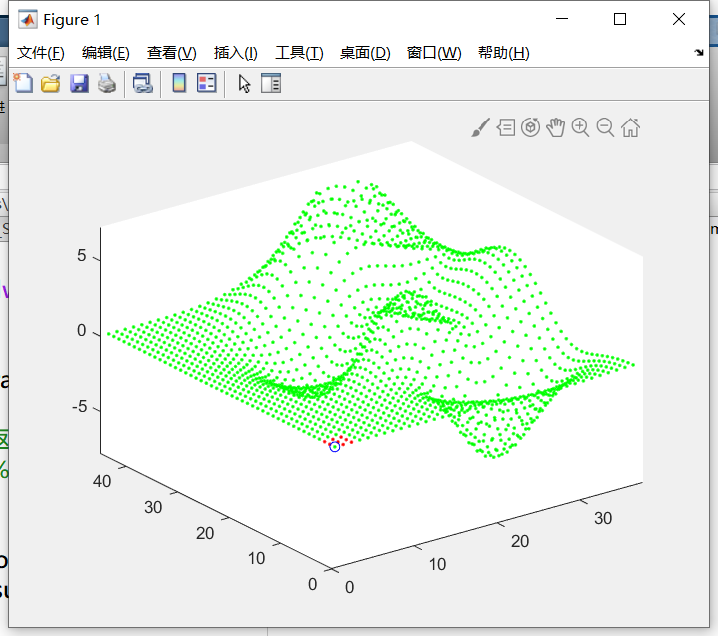使用手写的PCA计算出的法向量和matlab点云处理工具箱计算的法向量的效果对比如下，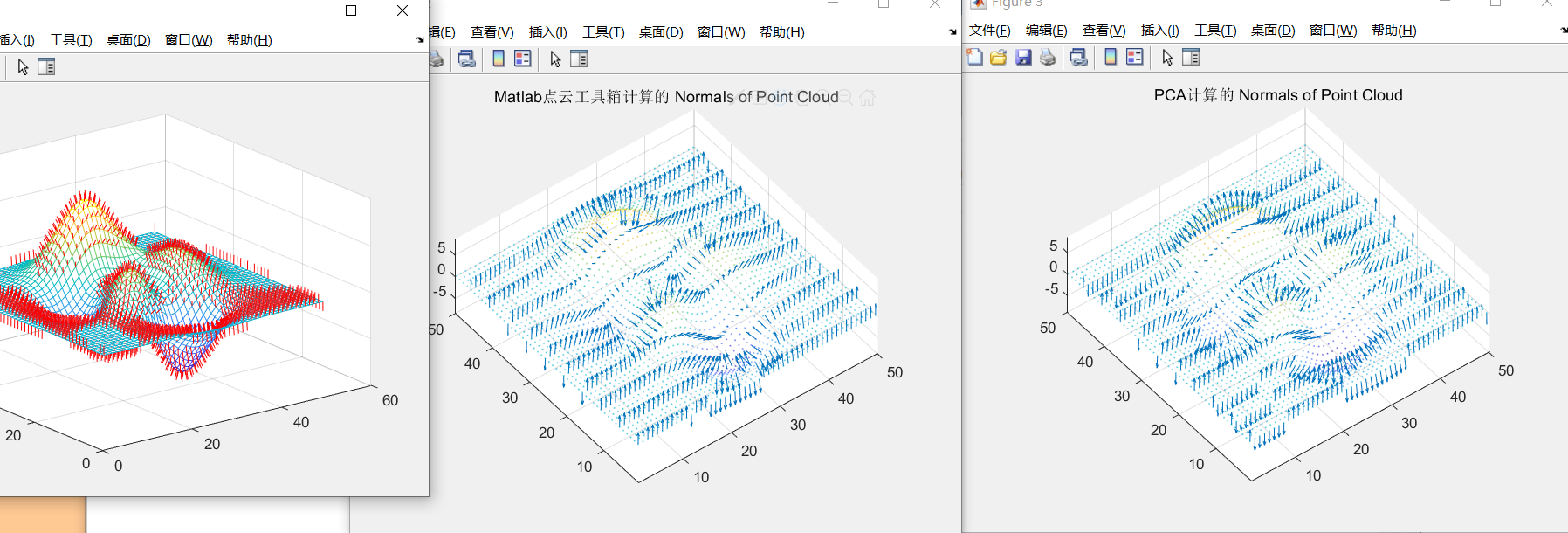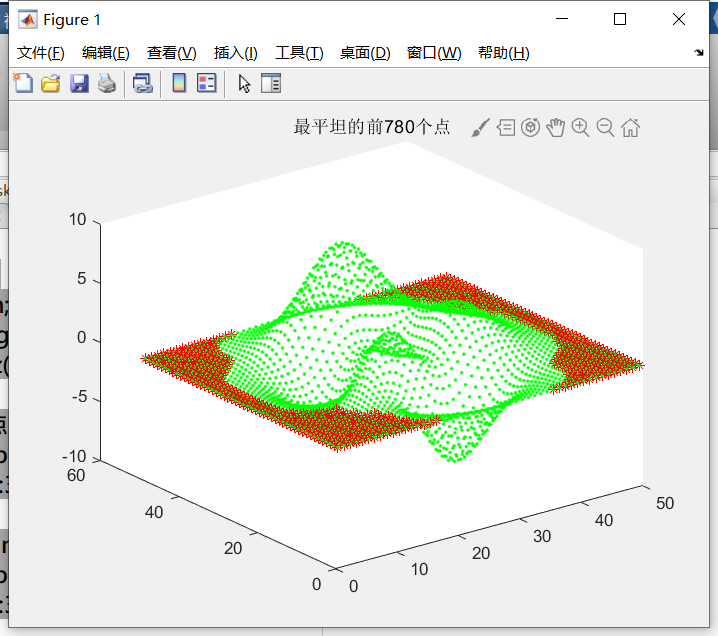可以看到，基本上是一样的。


展开全文matlab 点云 pca
• 因为做点云处理，在工作中接触到了PCA。 主要遇到的用法有两个：一个是对无预知信息的散乱点云进行向量重建；另外一个是对两片散乱点云进行配准时通过PCA来校准主方向。 学习笔记。。
因为做点云处理，在工作中接触到了PCA。
主要遇到的用法有两个：一个是对无预知信息的散乱点云进行法向量重建；另外一个是对两片散乱点云进行配准时通过PCA来校准主方向。
因为接触到的论文都言之不详，一直以来我只了解到使用PCA确定主方向的步骤：建立协方差矩阵，矩阵最大特征值对应的特征向量就是主方向。然而为什么要这么做，这么做是有着怎么样的物理背景，直到前些天专门去啃了PCA相关原理才清楚。
了解到具体原理的时候，我共有两个感想：一个是矩阵分析真是一门有用的公共课；第二个是头一次发现矩阵分析居然真的有用。。
PCA的原理，就以点云为例。点云是一系列有着(x,y,z)坐标的离散点，当然图像处理的时候很多特征向量也可以当作若干维(如128维的sift特征)特征空间中的离散点云来处理。如果一幅点云中有n个点，那么可以将点云信息写成3xn的矩阵，其中矩阵的每一列表示一个点。
矩阵的旋转是通过对数据矩阵左乘一个3x3的标准正交矩阵完成的，这种做法的实际意义在于：它是一种基变换！由一组标准正交基变换到另一组标准正交基，点云中点的相对位置没有发生改变，只是点云整体被旋转了一个角度。PCA就是基于这种“旋转”来设计的。
PCA对数据进行“旋转”，目的是为了降维，为了删除掉一些影响比较小的维度。而判断影响大小的参数是信噪比，即空域(或特征域)低频分量与高频分量之比(用方差评估频率，方差之比)。空域信息处于较高频的维度被认为是噪声，空域表现为点在该坐标轴上的投影分布较为集中，方差较小；空域信息处于较低频的维度被认为是信号，空域表现为点在该坐标轴上的投影分布较发散，方差较大。所以用于降维的PCA实际上是一个低通滤波器。
对于符合高斯分布的一般数据来说，可以用它们之间的协方差来表示相关性的大小。那么，对于点云在x维度上的数据和在y轴上的数据求取协方差，若协方差很小，说明两组数据较为独立，这两个维度能够储存最多的信息；若协方差很大，说明两组数据高度相关，这两个维度表示的信息高度重复。而对于点云数据的协方差矩阵来说，它非主对角线上的元素正是各维度之间的协方差，主对角线上的元素代表着该维度下数据的方差(即信息量)。于是，可以通过基变换使矩阵化为对角阵，在新的标准正交基下，数据在各个维度上的投影之间相关度达到最小。此时，各个维度上信息的丰富程度(分辨率)可以由主对角线上的元素（协方差矩阵的特征值）线性表征。协方差矩阵各特征值对应的特征向量即为新的标准正交基的一个基向量，而基向量的各个维度下信息的丰富程度是与特征值线性相关的。所以可以用最大特征值对应的特征向量来确定多维数据的主元，所以可以对基变换以后的数据删除一部分分辨率较低的维度来实现降维。
推论：PCA对于离群点很敏感；PCA直接计算出的主元是不分方向或者随机分配方向的。


展开全文点云 PCA
• 主元分析法在过程监控中的应用，陈晓智，，本文首先阐述了过程监控的研究对象和基本概念，从是否基于数学模型的角度介绍了目前存在的主要过程监控方法，并分析了它们的优缺
• 基于时变多块主元分析法的核电系统故障诊断，刘康玲，梁军，核电系统往往具有过程复杂、动态性和传感器变量庞多且高相关性等特点。主元分析法作为一种常用的降维技术，用于从众多的变量中提系统工程
• 核主成分分析算法，可以应用于过程监测和预警方面，计算SPE和T2指标进行表征。还可以进行降维。
• PCA主元分析法 做故障诊断程序 基于数据驱动的 也可以做图像处理PCA
• #include &lt;vtkAutoInit.h&gt; VTK_MODULE_INIT(vtkRenderingOpenGL); VTK_MODULE_INIT(vtkInteractionStyle); VTK_MODULE_INIT(vtkRenderingFreeType); #include &lt;string&......
实现环境：pcl1.8.0+vs2015+win10
#include <vtkAutoInit.h>
VTK_MODULE_INIT(vtkRenderingOpenGL);
VTK_MODULE_INIT(vtkInteractionStyle);
VTK_MODULE_INIT(vtkRenderingFreeType);
#include <string>
#include <iostream>
#include <pcl/io/io.h>
#include <pcl/io/pcd_io.h>
#include <pcl/point_cloud.h>
#include <pcl/point_types.h>
#include <vector>
#include <pcl/visualization/pcl_visualizer.h>
#include <Eigen/Core>
#include <pcl/common/transforms.h>

using namespace std;
typedef pcl::PointXYZ PointType;
typedef pcl::Normal NormalType;

int main(int argc,char **argv)
{
pcl::PointCloud<PointType>::Ptr cloud(new pcl::PointCloud<PointType>());
pcl::PointCloud<NormalType>::Ptr cloud_normal(new pcl::PointCloud<NormalType>());

std::string fileName(argv);

Eigen::Vector4f pcaCentroid;
pcl::compute3DCentroid(*cloud, pcaCentroid);
Eigen::Matrix3f covariance;
pcl::computeCovarianceMatrixNormalized(*cloud, pcaCentroid, covariance);
Eigen::Matrix3f eigenVectorsPCA = eigen_solver.eigenvectors();
Eigen::Vector3f eigenValuesPCA = eigen_solver.eigenvalues();
//eigenVectorsPCA.col(2) = eigenVectorsPCA.col(0).cross(eigenVectorsPCA.col(1));

Eigen::Matrix4f transform(Eigen::Matrix4f::Identity());
transform.block<3, 3>(0, 0) = eigenVectorsPCA.transpose();
transform.block<3, 1>(0, 3) = -1.0f * (transform.block<3,3>(0,0)) * (pcaCentroid.head<3>());//

pcl::PointCloud<PointType>::Ptr transformedCloud(new pcl::PointCloud<PointType>);
pcl::transformPointCloud(*cloud, *transformedCloud, transform);

std::cout << eigenValuesPCA << std::endl;
std::cout << eigenVectorsPCA << std::endl;

//转换到原点时的主方向
PointType o;
o.x = 0.0;
o.y = 0.0;
o.z = 0.0;
Eigen::Affine3f tra_aff(transform);
Eigen::Vector3f pz = eigenVectorsPCA.col(0);
Eigen::Vector3f py = eigenVectorsPCA.col(1);
Eigen::Vector3f px = eigenVectorsPCA.col(2);
pcl::transformVector(pz, pz, tra_aff);
pcl::transformVector(py, py, tra_aff);
pcl::transformVector(px, px, tra_aff);
PointType pcaZ;
pcaZ.x = 1000 * pz(0);
pcaZ.y = 1000 * pz(1);
pcaZ.z = 1000 * pz(2);
PointType pcaY;
pcaY.x = 1000 * py(0);
pcaY.y = 1000 * py(1);
pcaY.z = 1000 * py(2);
PointType pcaX;
pcaX.x = 1000 * px(0);
pcaX.y = 1000 * px(1);
pcaX.z = 1000 * px(2);

//初始位置时的主方向
PointType c;
c.x = pcaCentroid(0);
c.y = pcaCentroid(1);
c.z = pcaCentroid(2);
PointType pcZ;
pcZ.x = 1000 * eigenVectorsPCA(0, 0) + c.x;
pcZ.y = 1000 * eigenVectorsPCA(1, 0) + c.y;
pcZ.z = 1000 * eigenVectorsPCA(2, 0) + c.z;
PointType pcY;
pcY.x = 1000 * eigenVectorsPCA(0, 1) + c.x;
pcY.y = 1000 * eigenVectorsPCA(1, 1) + c.y;
pcY.z = 1000 * eigenVectorsPCA(2, 1) + c.z;
PointType pcX;
pcX.x = 1000 * eigenVectorsPCA(0, 2) + c.x;
pcX.y = 1000 * eigenVectorsPCA(1, 2) + c.y;
pcX.z = 1000 * eigenVectorsPCA(2, 2) + c.z;
//visualization
pcl::visualization::PCLVisualizer viewer;
pcl::visualization::PointCloudColorHandlerCustom<PointType> color_handler(cloud,255, 0, 0);
pcl::visualization::PointCloudColorHandlerCustom<PointType> tc_handler(transformedCloud, 0, 255, 0);

viewer.addArrow(pcaZ, o, 0.0, 0.0, 1.0, false, "arrow_Z");
viewer.addArrow(pcaY, o, 0.0, 1.0, 0.0, false, "arrow_Y");
viewer.addArrow(pcaX, o, 1.0, 0.0, 0.0, false, "arrow_X");

viewer.addArrow(pcZ, c, 0.0, 0.0, 1.0, false, "arrow_z");
viewer.addArrow(pcY, c, 0.0, 1.0, 0.0, false, "arrow_y");
viewer.addArrow(pcX, c, 1.0, 0.0, 0.0, false, "arrow_x");

viewer.setBackgroundColor(1.0, 1.0, 1.0);
while (!viewer.wasStopped())
{
viewer.spinOnce(100);

}

return 0;
}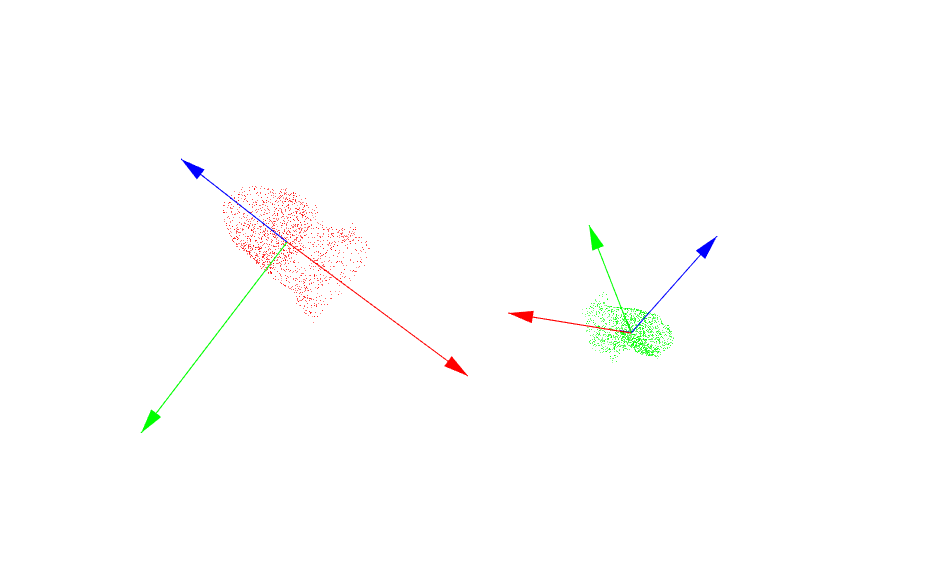展开全文pca
• PCA，即主元分析法，是用来做数据降维的一种方法。从思想上讲，一个高纬的数据，往往包含很多多余的信息，我们希望将其取出，尽可能地降到一个较低的维度，但同时又必须保证其数据失真尽可能小。PCA就是一套算法，在...
PCA，即主元分析法，是用来做数据降维的一种方法。从思想上讲，一个高纬的数据，往往包含很多多余的信息，我们希望将其取出，尽可能地降到一个较低的维度，但同时又必须保证其数据失真尽可能小。PCA就是一套算法，在保证重建误差最小的情况下，提取出高维数据的主元，从而实现数据的降维。  下面具体来说PCA算法在人脸识别中的算法流程，前提：有一个训练图集，里面放着同一个人的脸（对齐过的），并且每张图长宽的像素点都相同（比如160*120）；有一个测试图集，里面放着待测图像，待测图像的长宽像素点与训练图集一样。  PCA的算法流程是：  一、训练流程  S1：构筑训练图像矩阵：将每一张人脸图张开成一维行向量（也可以是列向量，但以下的计算都是用行向量），训练集中所有图像组成一个训练图像矩阵，每一个行向量代表一张图。  S2：计算平均脸、协方差矩阵：平均脸就是将所有的行向量求平均得到的一个平均的行向量，将每张脸向量按下面公式计算得到协方差矩阵。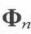S3：计算协方差矩阵的特征值与特征向量，取前r大的特征值对应的特征向量构成r维特征空间，r
展开全文脸部识别
• ## 如何理解主元分析（PCA）？

万次阅读 多人点赞 2018-08-31 12:14:03
主元分析也就是PCA，主要用于数据降维。 1 什么是降维？ 比如说有如下的房价数据： 这种一维数据可以直接放在实数轴上： 不过数据还需要处理下，假设房价样本用 表示，那么均值为： 然后以均值 为原点...pcaPCA 机器学习
• 介绍了主元分析的原理，主元分析的应用。在matlab中如何实现
• PCA，即主元分析法，是用来做数据降维的一种方法。从思想上讲，一个高纬的数据，往往包含很多多余的信息，我们希望将其取出，尽可能地降到一个较低的维度，但同时又必须保证其数据失真尽可能小。PCA就是一套算法，在...人脸识别 算法 测试 编程
• 主元分析法,PCA，EMG信号主元提取，降低噪声
• 用MATLAB实现部分主元法的LU分解，选取列中绝对值最大的行实现行交换。
• PCA（Principal Component Analysis）是一种常用的数据分析方法。PCA通过线性变换将原始数据变换为一组各维度线性无关的表示，可用于提取数据的主要特征分量，常用于高维数据的降维。网上关于PCA的文章有很多，但是...
• 本文首先介绍了故障诊断方法、多元统计方法和TE过程模型，研究了基于主元 ...，将动态主元分析用于故障检测与诊断，提出基于动态主元分析的故障检测与诊断 方法，以TE模型为对象进行了仿真研究和仿真结果分析。主
• 正如它的名字：主元分析，这种方法可以有效的找出数据中最“主要”的元素和结构，去除噪音和冗余，将原有的复杂数据降维，揭示隐藏在复杂数据背后的简单结构。它的优点是简单，而且无参数限制，可以
• 提出一种基于保留最佳主成分的主元分析法(PCA)和整数小波变换相结合的超光谱图像压缩新方法, PCA能够有效地消除超光谱图像的谱间相关性, 而整数小波变换在去除空间相关性方面具有长处。对超光谱图像的空间进行等份...
• 本文从基于数据驱动的故障诊断方法出发，主要研究了动态主元分析(DPCA)算 离线和在线的自适应故障诊断过程，本文的主要工作和贡献如下： (1)阐述了故障诊断的研究现状，并从定性分析和定量分析角度研究了故障...
• 在matlab上实现kpca的程序，没有任何问题，绝对可以运行kpca...